# Understanding Steering Behaviors: WanderThis post is part of a series called Understanding Steering Behaviors.
Understanding Steering Behaviors: Flee and Arrival
Understanding Steering Behaviors: Pursuit and Evade

Steering behaviors aim to help autonomous characters move in a realistic manner, by using simple forces that combine to produce life-like, improvisational navigation. In this tutorial, I'll cover the wander behavior, which makes the character move randomly.

Note: Although this tutorial is written using AS3 and Flash, you should be able to use the same techniques and concepts in almost any game development environment. You must have a basic understanding of math vectors.

## Wandering Around

Often the characters of a game must randomly move around their environment. Usually those characters are just waiting for something to happen (like a battle against the player) or they are looking for something. When the player is able to see that behavior, the character's wandering ability must be visually pleasant and realistic enough.

If the player is able to identify strongly defined path lines or unrealistic move behavior, it will generate frustration. In the worst case the player will figure out how to anticipate the character's movements, which results in a boring game experience.

The wander steering behavior aims to produce a realistic "casual" movement, which will make the player think that the character is really alive and walking around.

## Seek and Randomness

There are several ways to implement the wander pattern using steering behaviors. The simplest is using the previously described seek behavior. When a character is performing seek, it will move towards a target.

If the position of that target changes every few seconds, then the character will never be able to reach the target (and even if it does,  the target will move again). Placing the target randomly in the game area will make the character eventually move around the whole environment chasing the target. The demo below shows this approach in action:

The seek behavior and a random target. Click the demo to show force vectors.

The code for this implementation would be:

 1 2 // Calculate the wander force  3 private function wander() :Vector3D {  4  var now :Number = (new Date()).getTime();  5 6  if (now >= nextDecision) {  7  // Choose a random position for "target"  8  }  9 10  // return a steering force that pushes the character  11  // towards the target (the seek behavior)  12  return seek(target);  13 }  14 15 // In the game loop, handle forces and movement just  16 // like before:  17 public function update() :void {  18  steering = wander()  19  steering = truncate (steering, max_force)  20  steering = steering / mass  21  velocity = truncate (velocity + steering , max_speed)  22  position = position + velocity  23 } 

Even though this is a simple and good approach, the final result is not so convincing. Sometimes the character completely inverts its route because the target is placed behind it. The character's behavior seems much more like "Damn, I forgot my keys!" than "Okay, I will follow this direction now".

## Wander

Another implementation of the wander behavior was proposed by Craig W. Reynolds when he invented those behaviors. The basic idea is to produce small random displacements and apply to the character's current direction vector (the velocity, in our case) every game frame. Since the velocity vector defines where the character is heading to and how much it moves every frame, any interference with that vector will change the current route.

Using small displacements every frame prevents the character from abruptly changing its route. If the character is moving up and turning right, for instance, in the next game frame it will still be moving up and turning right, but at a slightly different angle.

That approach can also be implemented in different ways. One of them is to use a circle in front of the character, using that to calculate all forces involved: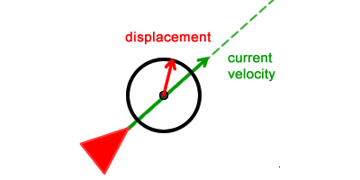The displacement force  has its origin at the circle's center, and is constrained by the circle radius. The greater the radius and the distance from character to the circle, the stronger the "push" the character will receive every game frame.

This displacement force will be used to interfere with the character's route. It is used to calculate the wander force.

## Calculating the Circle's Position

The first component required to calculate the wander force is the circle's center position. Since the circle should be placed in front of the character, the velocity vector can be used as a guide:

 1 2 // The CIRCLE_DISTANCE constant below is  3 // a number defined somewhere else.  4 // The code to calculate the circle center:  5 var circleCenter :Vector3D;  6 circleCenter = velocity.clone();  7 circleCenter.normalize();  8 circleCenter.scaleBy(CIRCLE_DISTANCE); 

The circleCenter vector above is a clone (copy) of the velocity vector, which means they point to the same direction. It is normalized and multiplied by a scalar value (CIRCLE_DISTANCE, in this case) which will result in the following vector: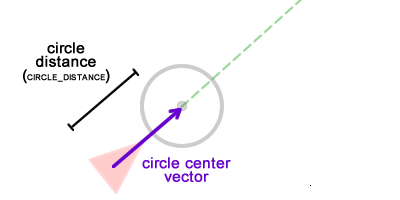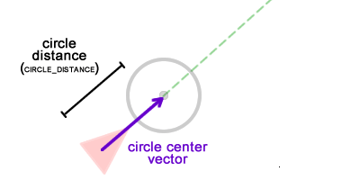## Displacement Force

The next component is the displacement force, which is responsible for the right or left turn. Since this is a force used to produce disturbance, it can point anywhere. Let's use a vector aligned with the Y axis:

 1 2 var displacement :Vector3D;  3 displacement = new Vector3D(0, -1);  4 displacement.scaleBy(CIRCLE_RADIUS);  5 //  6 // Randomly change the vector direction  7 // by making it change its current angle  8 setAngle(displacement, wanderAngle);  9 //  10 // Change wanderAngle just a bit, so it  11 // won't have the same value in the  12 // next game frame.  13 wanderAngle += (Math.random() * ANGLE_CHANGE) - (ANGLE_CHANGE * .5); 

The displacement force is created and scaled by the circle radius. As previously described, the greater the radius, the stronger the wander force. The wanderAngle is a scalar value that defines how much the displacement force should be "tilted"; after it is used a random value is added to make it different for the next game frame. It produces the required randomness in the movement.

For the sake of understanding, let's assume the displacement force calculated above is placed at the circle's center. Since it was scaled by the circle radius value, it would be something like this: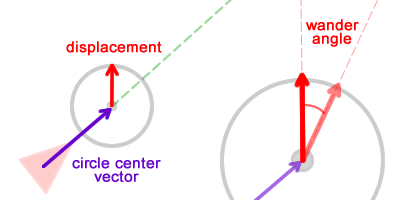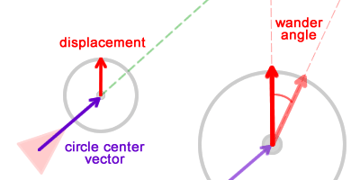Tip: Remember that math vectors do not have a position in the space, they have a direction and a magnitude (length). As a consequence they can be placed anywhere.

## Wander Force

After the circle center and the displacement vector are calculated, they must be combined to produce the wander force. That force is calculated by adding those two vectors:

 1 2 var wanderForce :Vector3D;  3 wanderForce = circleCenter.add(displacement); 

We can visually represent these forces like so: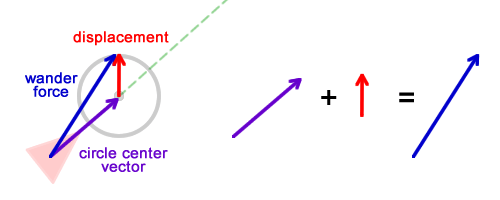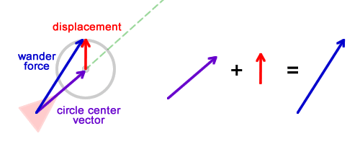The wander force can be imagined as a vector that goes from the character to a point on the circle's circumference. Depending on the position of that point, the wander force will push the character to the left or to the right, strongly or weakly: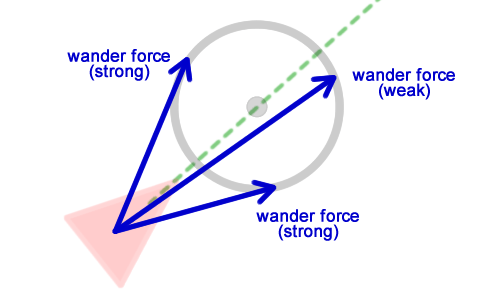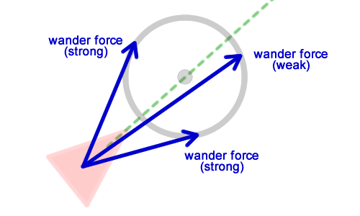The more the wander force is aligned with the velocity vector, the less the character will change its current route. The wander force will work exactly like the seek and flee forces: it will push the character towards a direction.

Similar to seek and flee where the force direction is calculated based on a target, the wander direction is calculated based on a random point on the circle's circumference. The final code for the wander force is:

 1 2 private function wander() :Vector3D {  3  // Calculate the circle center  4  var circleCenter :Vector3D;  5  circleCenter = velocity.clone();  6  circleCenter.normalize();  7  circleCenter.scaleBy(CIRCLE_DISTANCE);  8  //  9  // Calculate the displacement force  10  var displacement :Vector3D;  11  displacement = new Vector3D(0, -1);  12  displacement.scaleBy(CIRCLE_RADIUS);  13  //  14  // Randomly change the vector direction  15  // by making it change its current angle  16  setAngle(displacement, wanderAngle);  17  //  18  // Change wanderAngle just a bit, so it  19  // won't have the same value in the  20  // next game frame.  21  wanderAngle += Math.random() * ANGLE_CHANGE - ANGLE_CHANGE * .5;  22  //  23  // Finally calculate and return the wander force  24  var wanderForce :Vector3D;  25  wanderForce = circleCenter.add(displacement);  26  return wanderForce;  27 }  28 29 public function setAngle(vector :Vector3D, value:Number):void {  30  var len :Number = vector.length;  31  vector.x = Math.cos(value) * len;  32  vector.y = Math.sin(value) * len;  33 } 

After the wander force has been calculated, it must be added to the character's velocity so it can affect its movement. The addition of that force is performed in the same way as before:

 1 2 steering = wander()  3 steering = truncate (steering, max_force)  4 steering = steering / mass  5 velocity = truncate (velocity + steering , max_speed)  6 position = position + velocity 

The wander force will affect the character's route in the same way that the previously described behaviors did, with the difference that it pushes the character to a random direction each game frame:

The wander behavior. Click the demo to show force vectors.

The demo above shows several characters wandering in the environment. If they leave the screen they are respawned at the center.

## Conclusion

The wander behavior is an excellent way of implementing random movements. It is controlled by an imaginary circle placed in front of the character, which can be tweaked to produce the desired movement pattern.

This tutorial described steering behaviors, explaining the wander behavior. In the next post, we will learn about more behaviors. Keep up to date by following us on Twitter, Facebook, or Google+.